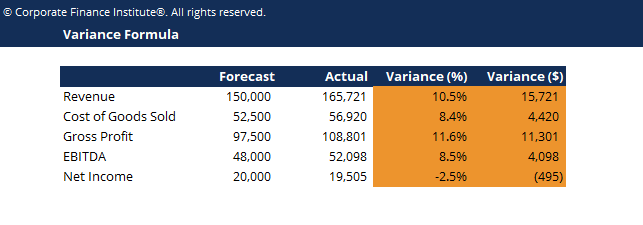# Variance Formula Template

## Variance Formula Template

This variance formula template helps you find out the variance between actual and forecast financial figures.

This is what the variance formula template looks like:### Variance Formula Template

The variance formula is used to calculate the difference between a forecast and the actual result. The variance can be expressed as a percentage or an integer (dollar value or the number of units).  Variance analysis and the variance formula play an import role in corporate financial planning and analysis (FP&A) to help evaluate results and make informed decisions for the business going forward.

There are two formulas to calculate variance:

Variance % = Actual / Forecast – 1  or  Variance \$ = Actual – Forecast

### Variance in budgeting and forecasting

The variance formula is useful in budgeting and forecasting when analyzing results.  The job of a financial analyst is to measure results, compare them to the budget/forecast, and explain what caused any difference.

In the Financial Planning & Analysis department at a company, the role of FP&A is to present management with accurate, timely, and insightful information so they can make effective decisions about the business going forward.

### More Free Templates

For more resources, check out our business templates library to download numerous free Excel modeling, PowerPoint presentation and Word document templates.

• Excel Modeling Templates
• PowerPoint Presentation Templates
• Transaction Document Templates

### Financial Analyst Certification

Become a certified Financial Modeling and Valuation Analyst (FMVA)® by completing CFI’s online financial modeling classes and training program!